# A Hybrid Method Based on LSTM and Optimized SVM for Diagnosis of Novel Coronavirus (COVID-19)

A Hybrid Method Based on LSTM and Optimized SVM for Diagnosis of Novel Coronavirus (COVID-19)
Department of Computer Engineering and Information Technology, Razi University, Kermanshah 94171-71946, Iran

Corresponding Author Email:
j.m.challab@razi.ac.ir
Page:
1061-1069
|
DOI:
https://doi.org/10.18280/ts.380416
22 July 2021
|
Accepted:
19 August 2021
|
Published:
31 August 2021
| Citation

OPEN ACCESS

Abstract:

The coronavirus disease of 2019 (COVID-19), a unique Coronavirus strain, has created a chaotic situation, negatively impacting the number of deaths and people's lives globally. The daily increase in COVID-19 instances is due to a lack of and restricted availability of detection techniques for determining the disease's presence. Therefore, detecting positive results as soon as feasible is important to preventing the spread of this epidemic and treating infected people as soon as possible. As a result of these constraints, the demand for clinical decision-making systems based on predictive algorithms has increased. The article describes a recurrent neural network (RNN) for identifying Coronavirus (COVID-19) and tries to improve the detection method. Different machine learning methodologies, such as Support Vector Machines (SVMs), were used to create a detection system with a deep learning algorithm called Long Short Term Memory (LSTM). The research describes a method for detecting COVID-19 in tagged CT images of patients. Various common picture features, such as central moments, Gabor wavelets, and GLCM-related features, are discussed. Ant colony optimization-ant lion optimization (ACO-ALO) is used to select optimum subsets of SVM parameters. The results show that SVM parameters such as penalty and kernel parameters have a positive effect on SVM model correctness and complexity. Besides, the findings revealed that the proposed method may be employed as a system of aid to diagnose COVID-19 disease. The findings uncover that the suggested strategy has promising behavior in terms of increasing classification accuracies as well as optimal feature selection. Promisingly, the presented strategy can be regarded as a useful clinical decision-making tool for clinicians.

Keywords:

ant colony optimization (ACO), COVID-19, ant lion optimization (ALO), support vector machine (SVM), RNN

1. Introduction

COVID-19, a unique type of coronavirus, has led to the chaos that has affected the number of fatalities and lives worldwide. This first appeared in Wuhan, China, in December 2019. This has spread to approximately 200 countries worldwide. Many governments and rulers in many countries have pondered unique tactics and adopted unique lifestyles to prevent COVID-19. Today's science and technology have made a significant contribution to the execution of such novel state policies in an unpredictable and unknow n process .

Typical COVID-19 clinical features contain breath shortness, muscle pain, headache, cough, fever, sore throat, fatigue . Screening workstations restricted availability as well as testing kits for detecting COVID19 makes the tremendous burden for medical staffs as well as professionals for controlling situation. In this respect, accurate and quick COVID-19 detection suspected cases is the great challenge for medical professionals. Cases exponential boost arises multiple testing needs for getting an opinion on accurate situation also in accordingly creating the suitable decision. Early COVID-19 detection suspected cases is the challenge while this comes to public security of health as well as pandemic control. Each failure in COVID-19 virus detection induced results of disease in increasing the rate of mortality. Period of incubation that means time among getting virus as well as starting for having disease signs is 1-14 days. It makes this more difficult for detecting disease of COVID-19 at too early stage according to signs which are illustrated by an individual .

Due to all such restrictions, the artificial intelligence (AI) helped system is needed for making these decisions. AI is utilized in systems of healthcare actively for presenting clinical support of decision. Classifiers of Machine learning are useful for interpreting medical findings like diseases of muscles, nerve, and epilepsy as well as heart rhythms. Algorithms of Deep learning are also useful for predicting the clinical findings from biomedical studies, virus diseases as well as cancers. These techniques are effective also they are able to be utilized for predicting infection of COVID-19.

SVM is one of the popular supervised algorithms of machine learning that is able to be utilized for the both challenges of classification and regression. SVM algorithm operation is according finding optimal hyper-plane (kernel) for discriminating among various levels. Parameters of SVM like parameters of kernel and penalty have the great effect on SVM model accuracy and complexity. Such parameters are usually selected randomly. But, SVM is highly required for determining optimal values of parameters for achieving the expected performance of learning. The proposed method is utilizing the new Ant lion hybrid metaheuristic algorithm based on Swarm  that integrates ant colony optimization (ACO) algorithm features  and the local algorithms of search are integrated with algorithm of ant lion optimization for optimizing the parameters before SVM.

The article describes how to use a recurrent neural network (RNN) to detect COVID-19 disease. The current investigation's goal is to create a detection system. To achieve the goal, various machine learning technologies, such as Support Vector Machines (SVMs), were used to build a detection system with a deep learning algorithm known as Long Short Term Memory (LSTM). Recurrent neural networks use blocks of long short-term memory to offer a context for how the program receives inputs and produces outputs.

To the best of our knowledge, the combination of both ACO and ALO techniques has not been attempted to optimize. In the present study, the ACO-ALO Hybrid algorithm combines the exploitation behavior of both ACO and ALO algorithms and has shown promising behavior in optimizing the parameters of SVM.

The current article structure is ordered as following. Related work is provided in part 2. Ant Colony Optimization is provided in part 3. Ant Lion Optimizer is provided in part 4. Detailed implementation of the proposed method will be defined in part 5. In part 6, we provide results and discussion after that conclude paper in part 7.

2. Related Work

AI has been taken as a potentially powerful tool in the fight against a lot of evolving pandemics like SARS (2002–2003), Middle East respiratory syndrome coronavirus (MERS-CoV) (2012-present), new coronavirus (COVID-19) (2019-ongoing), Ebola hemorrhagic fever (2014–2016), Swine flu (2002–2003). It has been realized that CT scans are the best images for diagnosing viruses in images. However, x-rays of the chest are able to be utilized for the same aim. It still contains good diagnostic value and are more available for people than getting CT scans, particularly in isolated as well as rural areas. As a recent example, we have papers below.

Jiang et al.  utilized the techniques of machine learning for predicting clinical coronavirus severity. Logistic regression was employed as well as the classifier’s performance was assessed with just the values of accuracy. de Moraes Batista et al.  used machine learning classifiers to predict COVID-19 diagnosis. Clinical data was obtained from the Israelita Albert Einstein Hospital in Sao Paulo, Brazil. Only five different classifiers were used: neural networks, SVM, gradient boosted trees, logistic regression, and random forests. Wang et al.  proposed a deep learning strategy based on the collection of 1065 CT scans, with the goal of enhancing the automated system that can perform clinical diagnosis. Bandyopadhyay and Dutta  presented a new method for automatically classifying negative, confirmed, and death cases using LSTM-GRU.

Khan et al.  developed a deep learning network for automatically predicting COVID-19 instances from chest X-rays. Yan et al.  developed a prognostic prediction model based on the XGBoost machine learning algorithm to predict mortality risk in patients before they are transferred to a critical case. Bullock et al.  analyzed datasets, tools, and resources required for AI research, as well as explored strategic aspects linked to the practical execution of multidisciplinary partnerships and open science. In South Korea, Al-Najjar and Al-Rousan  developed a classifier prediction model to forecast the state of recovered and dying coronavirus COVID-19 patients.

In the same regard, Pirouz et al.  revealed that the proposed binary classification model had a superior capability for predicting confirmed cases.

3. Ant Colony Optimization

ACO uses self-organizing concepts as well as the highly coordinated activity of real ants to recognize solutions to complex combinatorial optimization problems. Ants are social insects that live in colonies and have an unusual foraging activity. Ants can recognize the shortest path between a food source and their nest using Path Construction and Pheromone Update. Path construction: primarily, the ant moves on a random route in search of food sources. Walking deposits a chemical compound called a pheromone on the ground, which dissipates over time. As a result, shorter routes will contain significantly more pheromones than longer routes. Pheromone Update: Ants can smell pheromones and are drawn to shorter paths with higher concentrations of pheromones. Because more ants are drawn to shorter paths, pheromone levels rise in these areas. As a result, ants choose shorter routes (optimal solutions) while ignoring longer routes (non-optimal solutions). Such two phenomena keep driving algorithm of ACO to promising search space regions including solutions with high quality as shown in Figure 1.

## 1.png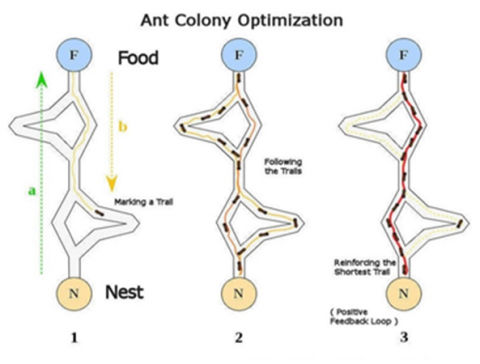Figure 1. Ant colony optimization 

4. Ant Lion Optimizer

Ant Lion Optimizer is a new optimization method that simulates Ant lion foraging behavior in nature. Antlions' random selection and ants' random travel around them ensure search space exploration in ALO, whereas adaptive antlions' traps reducing bounds ensure search space exploitation .

In the first step, the ALO algorithm creates an initial ant population and this means that a complete random solution set is created to solve the problem. The second step specifies whether the values given to the ant are accurate or not. In the third step, fitness function computation is done and the competency function of the ant shows how optimal this solution is.

ALO mathematical model is able to be defined with below steps help. Since ants stochastically move in nature while searching for food, a random ant's walk is able to be defined as below:

Xt = [0, cumsum(2r(t1) − 1), cumsum(2r(t2) − 1), ..., cumsum(2r(tn) − 1)]           (1)

Here Xt is random ants walk, n is iterations maximum number, cumsum provides cumulative sum, t is random walk step (iteration), r(t) is stochastic function which is described as below:

$r(t)= \begin{cases}1 & \text { if rand }>0.5 \\ 0 & \text { if rand } \leq 0.5\end{cases}$         (2)

That rand is random number created by uniform distribution in [0,1] interval (Figure 2). For keeping random ants walk in space of search, each ant position is normalized utilizing following min–max equation of normalization:

$X_{i}^{t}=\frac{\left(X_{i}^{t}-a_{i}\right)\left(b_{i}-c_{i}^{t}\right)}{d_{t}^{t}-a_{i}}$          (3)

## 2.png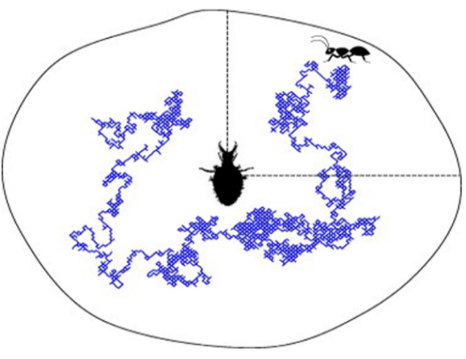Figure 2. An ant's random path within an antlion's trap

That ai is minimum random ith variable walk, bi is maximum random ith variable walk, $c_{\mathrm{i}}^{\mathrm{t}}$ is ith variable minimum at iteration t, $d_{\mathrm{i}}^{\mathrm{t}}$ is ith variable maximum at iteration t. By such mechanisms presented up to now, antlions can make traps which are proportional to the fitness of them and the ants are needed for randomly moving. In order to model the behavior mathematically, an ant’s random walk radius is adaptively reduced that is able to be defined as below:

$c^{t}=\frac{c^{t}}{I}$        (4)

$d^{t}=\frac{d^{t}}{I}$      (5)

That I = 10ω$\frac{t}{\mathrm{~T}}$, T is iterations maximum number, ω is constant which is described according to recent iteration (ω = 2 when t > 0.1T, ω = 3 while t > 0.5T, ω = 4 while t > 0.75T, ω = 5 while t > 0.9T, ω = 6 while t > 0.95T). Initially, constant ω is able to set exploitation accuracy level.

5. Proposed Method

Main aim is to focus on the proposed technique to group COVID-19 in either positive or negative. Basically, the proposed method contains 5 stages. Figure 3 shows the proposed system framework.

Image Acquisition, image Pre-processing, feature extraction, parameters optimization in SVM also classification step.

5.1 Acquisition of an image

In general, image acquisition is the process of obtaining images from various imaging modalities such as CT scans, MRI scans, and PET scans.

COVID-19 Input image is considered from slices of CT slices with suspected COVID-19 pneumonia signs that are marked by radiologists for each patient (positive, negative) in the paper to ensure that all patients’ images can be uploaded at capacity. Among them, images of CT (training) from a hospital that can be divided into validation, training as well as test datasets are saved in the "Data_For_Training_Validation_Test.hdf5" file. Each CT image shape section is (256, 256), down-sampled from (512, 512) to save space.

## 3.png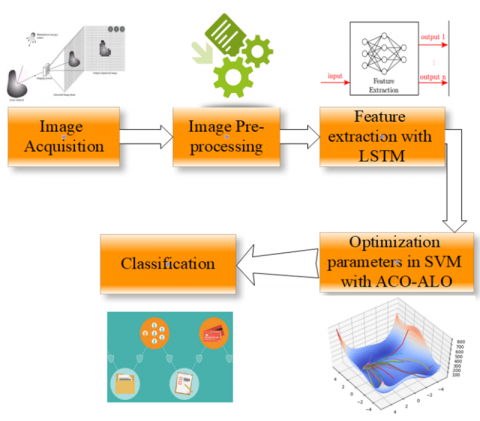Figure 3. Proposed system framework

Song et al.  conducted a retrospective study in which they enrolled 201 patients from two hospitals in China and found that 98 of them tested positive for COVID-19 (118 males and 83 females). The CT images of the hospitalized patient are shown in Figure 4.

## 4.png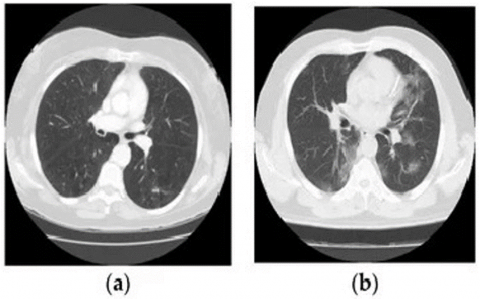Figure 4. CT images of the lungs obtained from the collected data: (a) Normal lung function and (b) COVID-19 infection

5.2 Image pre-processing

The basic goal of image enhancement and pre-processing is not to readily remove background information or undesired noise, but to change the quantities of image pixels. Histogram Equalization techniques are employed to improve images by utilizing the frequency and spatial field concepts. Most importantly, it improves the bounds of perception and image for human viewers. The most common frequency of filed techniques is histogram equalization, which is also employed to develop image contrast and is applicable for all image types.

5.3 Extraction of feature

It is possible to express the granted image as two different space variables function of y and x as f (x, y). Now, discrete values $i=0.1 \ldots . G-1$ can be considered by function f (x, y). G states all number of intensity levels in image. Pixels in all image which have intensity of:

$h(i)=\sum_{x=0}^{N-1} \sum_{y=0}^{M-1} \delta(f(x \cdot y) \cdot i)$        (6)

$\delta(j \cdot i)= \begin{cases}1 . & j=i \\ 0 . & j \neq i\end{cases}$        (7)

where, δ (j, i) states Kronecker delta function.

Central moments are achieved from it to characterize the texture as this is defined by following equations:

i. Mean:

$\mu=\sum_{i=0}^{G-1} i p(i)$       (8)

ii. Variance:

$\sigma^{2}=\sum_{i=0}^{G-1}(i-\mu)^{-2} p(i)$      (9)

iii. Skewness:

$\mu_{4}=\sum_{i=0}^{G-1}(i-\mu)^{-3} p(i)$          (10)

iv. Kurtosis:

$\mu_{4}=\sigma^{-4} \sum_{i=0}^{G-1}(i-\mu)^{-4} p(i)-3$           (11)

5.3.1 Features of texture

Gabor wavelets are used to acquire the texture feature. Manjunath and Ma proposed Gabor quality features for retrieval due to their superior performance in the simultaneous autoregressive model, which is multi-resolution, pyramid-structured, and tree-structured wavelet transmission features.

5.3.2 Grey-Level Co-occurrence Matrix (GLCM)

GLCM is a robust statistical approach for extracting textural second-order information from various images. GLCM is also known as the grey-level spatial dependence matrix. The majority of the process phases are (a) building a grey-Level Co-Occurrence Matrix, (b) setting Offsets, and (c) obtaining Statistics from GLCM. Statistics obtained include:

a) Contrast:

$\sum_{i=0}^{N_{p-1}} \sum_{j=0}^{N_{p-1}}(i-j)^{2} p(i \cdot j)$         (12)

b) Correlation:

$\sum_{\mathrm{i}=0}^{\mathrm{N}_{\mathrm{p}-1}} \sum_{\mathrm{j}=0}^{\mathrm{N}_{\mathrm{p}-1}} \frac{(\mathrm{i}-\mu)(\mathrm{j}-\mu) \mathrm{p}(\mathrm{i} \cdot \mathrm{j})}{\sigma_{\mathrm{i}} \sigma_{\mathrm{j}}}$                            (13)

c) Energy:

$\sum_{i=0}^{\mathrm{N}_{\mathrm{p}-1}} \sum_{\mathrm{j}=0}^{\mathrm{N}_{\mathrm{p}-1}} \mathrm{p}^{2}(\mathrm{i} . \mathrm{j})$                (14)

d) Homogeneity:

$\sum_{\mathrm{i}=0}^{\mathrm{N}_{\mathrm{p}-1}} \sum_{\mathrm{j}=0}^{\mathrm{N}_{\mathrm{p}-1}} \frac{\mathrm{p}(\mathrm{i} . \mathrm{j})}{1+|\mathrm{i}-\mathrm{j}|}$                               (15)

5.4 Long Short-Term Memory (LSTM)

A recurrent neural network employs blocks of LSTM to provide context for how a program receives inputs and also produces outputs. Of LSTM is a complicated unit with different elements, like activation functions, weighted inputs, inputs from eventual outputs as well as previous blocks .

Because the program's structure is based on short-term memory processes for creating longer-term memory, the unit is known as the block of long short-term memory. Often, such systems are utilized, for instance, in the natural processing of language. The Recurrent neural network uses blocks of LSTM to take specific phonemes/words and also evaluates this in other contexts in that the memory can be useful in categorizing and saving the types of such inputs . Long Short-Term Memory is widely accepted as well as a common concept in pioneering recurrent neural networks.

In the proposed method, LSTM is employed in the current study for the extraction of features. Here, the basic challenge is that we are not able to utilize Long Short-Term Memory as an extraction of a feature so easily. Because of that, the function is written well. These network layers are thus cut in such a way that they have been trained as needed (from the first layer to where we want to use them). We need up to a third layer, so we cut from the first to the third layer to create the novel network. These network layers are set up in such a way that we first take three layers that we cut, and then we take the regression layer (which just gives output). As a result, the LSTM is a recurrent network that cannot be used as an extraction system. As a result, we cut where the recurrent operation begins, and now we can perform feature extraction as a function of prediction. On the other hand, we created a network that looked like a normal network and also wanted to give us the regression output.

We utilized given features for training SVM. Due to that data are multi-class, we utilized the multi-class SVM. Also, we gave labeled data to SVM.

5.5 Hybrid algorithm of ACO-ALO

In this paper, the ACO-ALO Hybrid algorithm integrates the exploitation behaviour of both ACO and ALO algorithms and also demonstrates promising behaviour to optimize the parameter subset selection.

## 5.pngFigure 5. ACO

Therefore, we are able to choose parameters according to the performance of their classification . Locating these optimal parameters is too complicated and, therefore, techniques contain random/strategies of search with prohibitive complexity. In the literature, a lot of evolutionary and SI algorithms based methods such as BCO, PSO, GA, ACO, DE have been presented to optimize parameter problems. SI algorithm variants and hybrid forms have been tried for the optimization of parameters . The training dataset along with the parameters tuned by the ACO-ALO algorithm are used to train/learn SVM.

ACO stagnates in ALO, while intensifying. The ACO-ALO hybrid algorithm is presented in this manner; ACO only performs diversification, so there is no stagnation. The novel algorithm synergizes and synthesizes both ACO and ALO advantages. ACO explores feasible solutions accessible in the space of search and also creates solutions in the initial set. Bad solutions are filtered away and only the following generation ACO is given the best solutions. Now, ACO takes just the best solutions and also performs a global search for missing solutions. Both algorithms attempt to complement each other by hybridizing, with better intensification for delayed convergence and ACO avoidance for ALO.

The stages of parameter optimization with the ACO algorithm are shown in (Figure 5) and with the ALO algorithm are shown in (Figure 6). Such two algorithms form the proposed ACO-ALO hybrid algorithm motivation.

## 6.png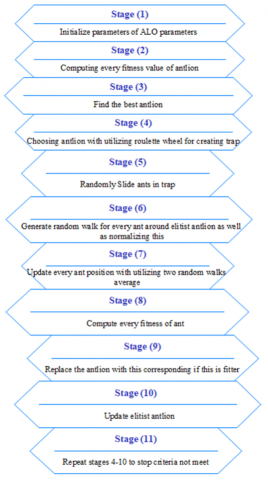Figure 6. ALO

5.5.1 Hybrid Algorithm of ACO-ABC

This section defines the detailed description of the proposed algorithm as well as the equations contained within it.

In essence, the populations of ants (K) in ACO, antlions (N), and ants in ALO are a set equal to (M). The entire set of parameters determines the pheromone values. The initialization phase is started first. The animals are then evaluated based on fitness function and ordered, with the best placed at the beginning, in the first repetition. In the following repetitions, a roulette wheel is used for each antlion, which is actually an antlion that wants to trap the ant with this antlion. Then, two random walks (RW) are performed. The average of these two RW is the new situation for ants. Then, we obtain the ants’ fitness. Indeed, the total population is calculated, which is the same as previous ants with ants; however, due to the updated situation, these new ants are combined with ants, as well as all fitness, before the ordering is performed. The first numbers will then be the new ones. Actually, in the ACO algorithm, we want to use the best ants obtained as the initial situation of ants in the ACO algorithm.

For ants in ACO in order to start search, every ant is determined subset of parameters including parameters random combination. Every ant chooses the probability based parameter as specified in Eq. (16):

$p=\tau_{\mathrm{t}} \cdot \Delta \tau_{\mathrm{t}}$        (16)

where, τι is parameter i pheromone value also i and ∆τι is ants proportion which have chosen the parameter.

Whenever the ant chooses the parameter, parameter pheromone value is updated by utilizing Eq. (17):

$\tau_{\mathrm{t}}=(1-\varphi) \cdot \tau_{\mathrm{t}}+\varphi \cdot \tau_{0}$           (17)

φ is relative importance parameter also takes values from 0-1.

After the whole ants have done a run, subset of parameters chosen by ants in ACO are utilized for SVM.

5.6 Classification with SVM

The Vector machine of support is a supervised learning algorithm that divides data into two groups. This is already trained with data series grouped into two categories, creating a model as it is trained initially. SVM algorithm task determines the group the novel point of data belongs to. It makes the SVM a -binary linear classifier type. The RBF kernel function is applied to the SVM in order to deal with high-dimensional data. The RBF kernel function was chosen because it performs better for high-dimensional classification problems with only two parameters, C and γ . The SVM parameters have a significant impact on the accuracy and complexity of the SVM model. Such parameters are usually selected randomly. But, SVM is highly required for determining optimal values of parameters for achieving the expected performance of learning. The proposed method utilizes the new Ant lion hybrid metaheuristic algorithm based on Swarm that integrates ant colony optimization (ACO) algorithm characteristics. Local search algorithms are also integrated with the ant and lion optimization algorithms for optimizing parameters prior to SVM.

6. Results and Discussion

Datasets utilized, implementation, and also the ACO-ALO results, are discussed in this part. The first stage is image acquisition. Now the input image is achieved from slices of CT with suspected COVID-19 pneumonia signs which are marked by the radiologists for every patient. After that, the image pre-processing and enhancement process is done. After taking the database, the image is read, then the resizing process is done. The database image is shown in Figure 7. It no abnormal findings on a CT scan of a 29-year-old female with the history of travel to Wuhan in the previous 14 days, and low fever and fatigue for 4 days, confirmed with COVID-19 positive.

## 7.png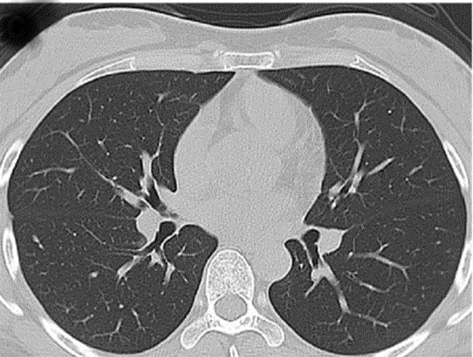Figure 7. CT image of the coronavirus disease 2019 (COVID-19 positive pneumonia patients)

6.1 Performance measures

MATLAB 2020b has been employed to implement the novel proposed method. To assess the outcomes, performance parameter measures are sensitivity, accuracy, precision, specificity, F1 score criteria. The criterion used to assess the efficacy of a method is determined by the problem that we are attempting to solve. By assuming there are data samples, which are fed into a model one by one, and a level is assigned to each of them as an output. A level which is predicted by model and data real class is able to be illustrated in Table 1. The Table is called the matrix of confusion.

Table 1. Confusion matrix

 Predicted class label Patient Healthy Predicted/real False positive (FP) True negative (TN) Healthy Real class label True positive (TP) False negative (FN) Patient
True positive: samples that are diagnosed accurately by test as patient.

False positive: samples that are diagnosed wrongly by test as patient.

True negative: samples that are diagnosed accurately by test as healthy.

False negative: samples that are diagnosed wrongly by test as healthy.

6.1.1 Criterion of accuracy

Accuracy is a test ability in healthy accurate dissociation as well as cases of patient from other cases. To calculate test accuracy, we must collect all true positive and true negative samples from all tested instances. The ratio can be expressed mathematically as follows:

Accuracy $=\frac{T P+T N}{T P+F P+F N+T N}$        (18)

6.1.2 Criterion of specificity

A covid19 percentage of accurate background can be discovered utilizing an automated method. The following is a definition of specificity:

Specificity $=\frac{T N}{T N+F P}$           (19)

6.1.3 Criterion of sensitivity

Criterion of Sensitivity is another criteria type for showing efficiency rules. This determines the patient's correct percentage of rule detection. If the amount is higher, the rules have a higher capability of detection.

ensitivity $=\frac{T P}{T P+F N}$       (20)

6.1.4 Criterion of precision

Precision is the other parameter which illustrates being patient prediction possibility as well as that prediction accuracy. If the amount is higher, this illustrates that this has more rules in detection of diseases.

Precision $=\frac{T P}{T P+F P}$       (21)

6.1.5 Criterion of F1 score

F1 score is described as sensitivity and precision harmonic average.

$F 1=\frac{2 \times T P}{2 T P+F P+F N}$        (22)

6.2 Initialization of parameters

Table 2. Basic values amount for parameters

 Parameters Values batch 100 trainPercent 0.50 testPercent 0.50 numHiddenUnits 100 maxEpochs 100 miniBatchSize 200 ρ 0.7 β 0.8 φ 0.3 $\tau_{0}$ 0.2 $\tau_{i}$ Multiple values varying with dataset numPopulation 10 Max_iter 10 lb 0.01 up 10

We adjust parameters, for assessing performance of proposed algorithm. Also, we assign data classification percentage to train and test equal to 80 and 20. We adjust basic values for parameters of LSTM (batch, numHiddenUnits, maxEpochs, miniBatchSize) and parameters of.ALO (numPopulation, Max_iter, lb, up) and parameters of ACO (ρ, β, φ, τ_0, τ_i). In Table 2, settings related to parameters are illustrated.

6.3 Results evaluation

After performing proposed method in environment of Matlab 2018b, we compared proposed method as well as other methods on dataset. Comparing these two methods results are given in Table 3.

In Table 3, we compare proposed algorithm performance results to the other criteria with the other methods on 1500 images dataset of COVID-19.

Based on the data in Table 3, it is possible to conclude the following:

(1) The proposed method of feature selection has significantly enhanced classification accuracy.

(2) When compared to state-of-the-art approaches, ACO-ALO provided the highest recognition rates for the dataset.

(3) In proposed method, there is 0.58% increase in the classification accuracy compared to method .

(4) In proposed method, the features selected are more optimal and has resulted in 7.38%. Improvement of sensitivity and 12.38% improvement of specificity in compared to method .

The search space is readily and efficiently reduced since the ants filter the redundant and undesired characteristics once, then by the utilized bees and the spectator bees. Table 3 additionally show that for the dataset, the proposed ACO-ALO –SVM approach outperforms the other techniques. The ACO-ALO-SVM algorithm has a fewer number of characteristics than other algorithms. The suggested ACO-ALO-SVM method achieves improved classification accuracy by identifying the relevant features that have a substantial influence on classification accuracy.

To evaluate the performance of ACO-ALO-SVM proposed method, 80% of the dataset is considered as a train set and 20% is considered as a test set. The accuracy of proposed method with epochs is depicted in Figure 8. For 1000 epochs the proposed system is trained. Fig.8 demonstrates how exact the proposed model is trained and tested at different times. Figure 9 additionally shows that the framework proposed achieves a general classification accuracy of 91.64%. Figure 9 shows the loss variation. This means that, as the number of training times grows, losses will reduce substantially.

This outcome could be due to the operations utilized by the ALO algorithm. When compared to the PSO and GA algorithms, the ALO method employs more onlooker bees. When a near-optimal solution is found, spectator bees might utilize it more in that region. As a result, it can more readily arrive at the best option. PSO algorithm and GA, on the other hand, lack this helpful feature. As a result, while they may be good at avoiding local optimal, they fail to do the deeper exploitation required in this situation.

Table 3. Proposed algorithm comparison results with the other methods

 Methods F1 score Precision Sensitivity Specificity Accuracy  - - 80 % 75% -  89.8% 90% 89.92% 96.4% 89.6% Proposed method 85.67% 84.27% 87.38% 87.38% 90.18%

## 8.pngFigure 8. Accuracy of the proposed method over epochs

## 9.png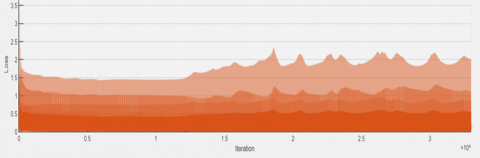Figure 9. Loss of the proposed method over epochs

## 10.png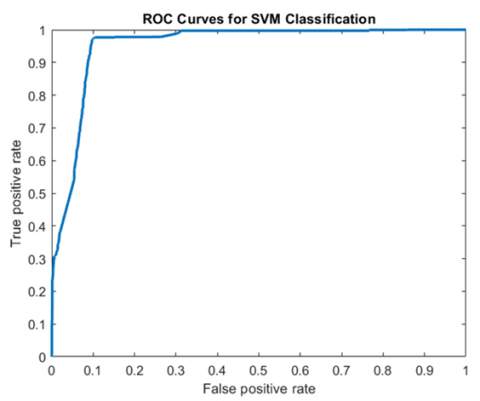Figure 10. The ROC curve for the proposed method

## 11.png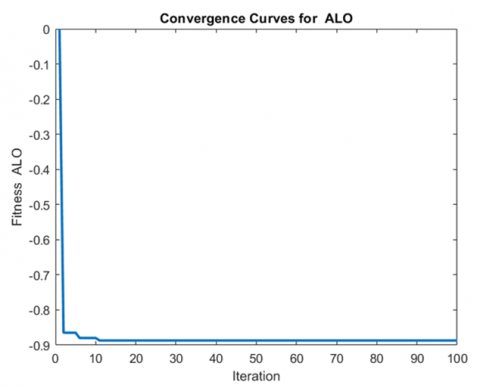Figure 11. Convergence curve for ALO

The curve for the suggested algorithm for reception operating properties (ROC) is presented in Figure 10. ROC reflects a true positive and false positive rate performance of the proposed algorithm, which is given a ROC of 0,99 by the proposed algorithm.

Figure 11 shows the convergence curve for the ALO, designed to give an optimum convergence. The ALO produces the greatest results and shows a greater potential for exploration from the outset, till a good parameter area is established. The main reasons are that antlion cones and roulette wheel selection have been filled with the ALO with a different degree of time.

7. Conclusions

As COVID-19 pandemic cases are constantly increasing, a lot of countries are facing resource shortages. During a health emergency, it is critical that not a single positive case goes unnoticed. By keeping this in mind, the current study offered a recurrent neural network (RNN) for detecting the COVID-19 disease. As a result, current research is aimed at improving the detection system. To achieve the goal, various machine learning approaches, such as SVMs, are used to build the detection system with a deep learning algorithm known as LSTM. The recurrent neural network uses blocks of LSTM to present context for how the program receives inputs and produces outputs. SVM is highly required to assign optimal values of parameters for obtaining the expected performance of learning. The proposed method utilizes a new Ant lion hybrid metaheuristic algorithm based on Swarm that integrates ant colony optimization (ACO) algorithm characteristics. Furthermore, the local search algorithms are integrated with the ant lion optimization algorithms for optimizing parameters prior to SVM. In addition, the network was successfully trained for both two classes of CT scan images (negative, positive) with a middle classification accuracy of 90.18% obtained using AC-ALO-SVM in comparison to the modern MATLAB 2018b software.

References

 Nour, M., Cömert, Z., Polat, K. (2020). A novel medical diagnosis model for COVID-19 infection detection based on deep features and Bayesian optimization. Applied Soft Computing, 97: 106580. https://doi.org/10.1016/j.asoc.2020.106580

 Ozturk, T., Talo, M., Yildirim, E.A., Baloglu, U.B., Yildirim, O., Acharya, U.R. (2020). Automated detection of COVID-19 cases using deep neural networks with X-ray images. Computers in Biology and Medicine, 121: 103792. https://doi.org/10.1016/j.compbiomed.2020.103792

 Jain, G., Mittal, D., Thakur, D., Mittal, M.K. (2020). A deep learning approach to detect COVID-19 coronavirus with X-ray images. Biocybernetics and Biomedical Engineering, 40(4): 1391-1405. https://doi.org/10.1016/j.bbe.2020.08.008

 Assiri, A.S., Hussien, A.G., Amin, M. (2020). Ant Lion Optimization: variants, hybrids, and applications. IEEE Access, 8: 77746-77764. https://doi.org/10.1109/ACCESS.2020.2990338

 Shunmugapriya, P., Kanmani, S. (2017). A hybrid algorithm using ant and bee colony optimization for feature selection and classification (AC-ABC Hybrid). Swarm and Evolutionary Computation, 36: 27-36. https://doi.org/10.1016/j.swevo.2017.04.002

 Jiang, X., Coffee, M., Bari, A., Wang, J., Jiang, X., Huang, J., Huang, Y. (2020). Towards an artificial intelligence framework for data-driven prediction of coronavirus clinical severity. Computers, Materials & Continua, 63(1): 537-551. https://doi.org/10.32604/cmc.2020.010691

 de Moraes Batista, A.F., Miraglia, J.L., Donato, T.H.R., Chiavegatto Filho, A.D.P. (2020). COVID-19 diagnosis prediction in emergency care patients: A machine learning approach. medRxiv.

 Wang, S., Kang, B., Ma, J., Zeng, X., Xiao, M., Guo, J., Xu, B. (2021). A deep learning algorithm using CT images to screen for Corona Virus Disease (COVID-19). European Radiology, 31: 6096-6104. https://doi.org/10.1007/s00330-021-07715-1

 Bandyopadhyay, S.K., Dutta, S. (2020). Machine learning approach for confirmation of COVID-19 cases: Positive, negative, death and release. MedRxiv. https://doi.org/10.1101/2020.03.25.20043505

 Khan, A.I., Shah, J.L., Bhat, M.M. (2020). CoroNet: A deep neural network for detection and diagnosis of COVID-19 from chest x-ray images. Computer Methods and Programs in Biomedicine, 196: 105581. https://doi.org/10.1016/j.cmpb.2020.105581

 Yan, L., Zhang, H.T., Xiao, Y., Wang, M., Guo, Y., Sun, C., Yuan, Y. (2020). Prediction of criticality in patients with severe COVID-19 infection using three clinical features: A machine learning-based prognostic model with clinical data in Wuhan. MedRxiv. https://doi.org/10.1101/2020.02.27.20028027

 Bullock, J., Luccioni, A., Pham, K.H., Lam, C.S.N., Luengo-Oroz, M. (2020). Mapping the landscape of artificial intelligence applications against COVID-19. Journal of Artificial Intelligence Research, 69: 807-845. https://doi.org/10.1613/jair.1.12162

 Al-Najjar, H., Al-Rousan, N. (2020). A classifier prediction model to predict the status of Coronavirus COVID-19 patients in South Korea. European Review for Medical and Pharmacological Sciences, 24: 3400-3403.

 Pirouz, B., Shaffiee Haghshenas, S., Shaffiee Haghshenas, S., Piro, P. (2020). Investigating a serious challenge in the sustainable development process: analysis of confirmed cases of COVID-19 (new type of coronavirus) through a binary classification using artificial intelligence and regression analysis. Sustainability, 12(6): 2427. https://doi.org/10.3390/su12062427

 Wikimedia, http://upload.wikimedia.org/wikipedia/commons/thumb/a/af/Aco_branches.svg/2000px-Aco_branches.svg.png, accessed on 11 August 2021.

 Assiri, A.S., Hussien, A.G., Amin, M. (2020). Ant Lion Optimization: Variants, hybrids, and applications. IEEE Access, 8: 77746-77764. https://doi.org/10.1109/ACCESS.2020.2990338

 Song, J., Wang, H., Liu, Y., Wu, W., Dai, G., Wu, Z., Deng, K. (2020). End-to-end automatic differentiation of the coronavirus disease 2019 (COVID-19) from viral pneumonia based on chest CT. European Journal of Nuclear Medicine and Molecular Imaging, 47(11): 2516-2524. https://doi.org/10.1007/s00259-020-04929-1

 Moldovan, D., Anghel, I., Cioara, T., Salomie, I. (2019). Time series features extraction versus LSTM for manufacturing processes performance prediction. 2019 International Conference on Speech Technology and Human-Computer Dialogue (SpeD), pp. 1-10. https://doi.org/10.1109/SPED.2019.8906653

 Van Houdt, G., Mosquera, C., Nápoles, G. (2020). A review on the long short-term memory model. Artificial Intelligence Review, 53(8): 5929-5955. https://doi.org/10.1007/s10462-020-09838-1

 Kuo, R.J., Huang, S.L., Zulvia, F.E., Liao, T.W. (2018). Artificial bee colony-based support vector machines with feature selection and parameter optimization for rule extraction. Knowledge and Information Systems, 55(1): 253-274. https://doi.org/10.1007/s10115-017-1083-8